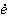### Create an Account

Already have account?

### Forgot Your Password ?

Home / Questions / Using the results of this problem obtain a relation for the volumetric heat generation rat...

# Using the results of this problem obtain a relation for the volumetric heat generation rate gen in Wm3 Then express the convection problem as an equivalent conduction problem in the oil layer

Using the results of this problem, obtain a relation for the volumetric heat generation rategen, in W/m3. Then express the convection problem as an equivalent conduction problem in the oil layer. Verify your model by solving the conduction problem and obtaining a relation for the maximum temperature, which should be identical to the one obtained in the convection analysis.

Problem. 6–61

Consider the flow of fluid between two large parallel isothermal plates separated by a distance L. The upper plate is moving at a constant velocity of V and maintained at temperature T0 while the lower plate is stationary and insulated. By simplifying and solving the continuity, momentum, and energy equations, obtain relations for the maximum temperature of fluid, the location where it occurs, and heat flux at the upper plate.

Jul 20 2020 View more View Less

#### Answer (Solved)Subscribe To Get Solution